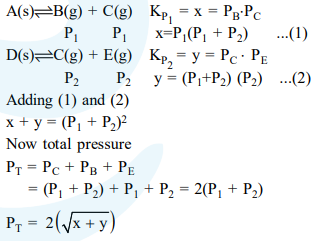# Two solids dissociate as follows

Question:

Two solids dissociate as follows

$\mathrm{A}(\mathrm{s}) \rightleftharpoons \mathrm{B}(\mathrm{g})+\mathrm{C}(\mathrm{g}) ; \mathrm{K}_{\mathrm{p}_{1}}=\mathrm{x} \mathrm{atm}^{2}$

$\mathrm{D}(\mathrm{s}) \rightleftharpoons \mathrm{C}(\mathrm{g})+\mathrm{E}(\mathrm{g}) ; \mathrm{K}_{\mathrm{p}_{2}}=\mathrm{y} a \mathrm{~atm}^{2}$

The total pressure when both the solids dissociate simultaneously is :-

1. $x^{2}+y^{2} a t m$

2. $x^{2}+y^{2}$ atm

3. $2(\sqrt{x+y}) a \operatorname{atm}$

4. $\sqrt{x+y}$ atm

Correct Option: , 3

Solution: